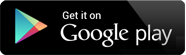## Video Lectures

Chapter 1 : Derivative and its Applications
Topic : Review of Basic Concepts, Functions, Limit of function, Continuity of function, Derivative function
Content : Composite Function, Classification of Function 64 MB ,47:7 MIN , THEORY

Chapter 1 : Derivative and its Applications
Topic : Derivative in Graphing and Applications, Increasing and Decreasing funtion
Content : Definition of increasing and decreasing function 36 MB ,25:26 MIN , THEORY

Chapter 1 : Derivative and its Applications
Topic : Concavity, Relative Extreme, Graphing Polynomials, Rational Functions
Content : Examples on Increasing and Decreasing function , Derivative of Rational function 52 MB ,37:25 MIN , THEORY

Chapter 1 : Derivative and its Applications
Topic : Cusps and vertical tangents, Absolute maxima and minima, Applied Maximum and minimum problems, Newton's method
Content : Local maxima and minima, Problems on Absolute maxima and Minima, Problem on Local maxima and minima 87 MB ,1:2:56 MIN , THEORY + PRACTICAL

Chapter 1 : Derivative and its Applications
Topic : More Examples on Differentiability, Increase and Decrease function, Concavity, Maxima and Minima
Content : Examples on Newton's Method 50 MB ,35:56 MIN , THEORY + PRACTICAL

Chapter 1 : Integration and its Applications
Topic : An overview of the Area Problem, Indefinite Integral
Content : Examples on Integration by parts, Examples on Substitution Method. 76 MB ,41:18 MIN , THEORY + PRACTICAL

Chapter 1 : Integration and its Applications
Topic : Definition of area as a Limit, Sigma notation, Definite Integral
Content : Summation formula, Properties of Definite Integral, Examples based on properties of Definite Integral 54 MB ,38:24 MIN , THEORY + PRACTICAL

Chapter 1 : Integration and its Applications
Topic : Evaluating Definite Integral by Substitution, Numerical Integration: Simpson's Rule, Application of Integration: Area between two curves, Length of a plane curve
Content : Examples based on Area between two curve, Example based on length of a plane curve 59 MB ,44:46 MIN , THEORY + PRACTICAL

Chapter 1 : Integration and its Applications
Topic : Mathematical Modeling with Differential Equations: Modeling with Differential Equations, Separation of Variables, Slope fields, Euler's Method, First-order Equations and Applications
Content : Newton's Law of Cooling, The Logistic Model, The Malthusian Model 95 MB ,1:8:49 MIN , THEORY

Chapter 1 : Integration and its Applications
Topic : Examples on Integration by parts, Substitution method, Simpson's rule, Separation of Variables, Euler's Method
Content : Examples on First Order Linear Differential Equation 22 MB ,17:29 MIN , THEORY + PRACTICAL

Chapter 1 : Partial Derivatives and its Applications
Topic : Functions of several variables: Functions of two or more variables, Limits and Continuity of functions of two or three variables, Partial derivatives , Differentiability
Content : First order partial derivative, Second order partial derivative 61 MB ,42:5 MIN , THEORY + PRACTICAL

Chapter 1 : Partial Derivatives and its Applications
Topic : Differentials and Local Linearity, Chain Rule, Implicit Differentiation, Directional Derivatives and Gradients
Content : Theorem on Directional Derivative and Gradient, Properties of Gradient Vector 82 MB ,55:12 MIN , THEORY + PRACTICAL

Chapter 1 : Partial Derivatives and its Applications
Topic : Applications of Partial Derivatives: Tangent planes and Normal vectors, Maxima and Minima of functions of two variables
Content : Problems on Maxima and Minima of functions of two variables 51 MB ,28:51 MIN , THEORY + PRACTICAL

Chapter 1 : Partial Derivatives and its Applications
Topic : Examples on Limits, Partial derivatives, Linearization, Chain rule, Implicit Differentiation, Theorem of Partial derivative, Gradient vector, Tangent and Normal
Content : Examples on Maxima and Minima 53 MB ,37:35 MIN , THEORY + PRACTICAL

## The Shikshak App

The Shikshak App is an honest attempt to provide quality education.